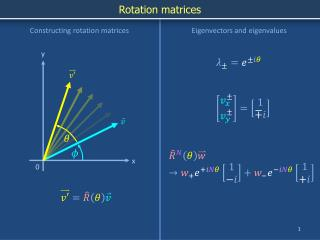DownloadDownload PresentationRotation matrices

# Rotation matrices

Download Presentation## Rotation matrices

- - - - - - - - - - - - - - - - - - - - - - - - - - - E N D - - - - - - - - - - - - - - - - - - - - - - - - - - -
##### Presentation Transcript

1. Rotation matrices Constructing rotation matrices Eigenvectors and eigenvalues y x 0

2. Rotating a vector y Length v x 0

3. Repeatedly rotating a vector y x 0 How can we recognize a rotation matrix? STOP

4. Rotation matrices Constructing rotation matrices Eigenvectors and eigenvalues y x 0

5. Complex eigenvalues and eigenvectors Please confirm these last 2 lines Complex eigenvalues Complex eigenvectors STOP

6. Complex eigenvalues and eigenvectors Complex eigenvalues Complex eigenvectors

7. Complex eigenvalues and eigenvectors y Consider an example with w+ = w- = w/2 x 0 In this example, the initial vector points directly to the right. How should the coefficients w+ and w- be changed to represent an initial vector pointing at an arbitrary initial angle relative to the x axis? Can you plot the eigenvectors (1, ±i) on the x-y axes? (No). Why not?